# Data science job market analysis

To better understand the market for data science jobs, I scraped 11,500 job ads from LinkedIn and explored the skills, tools, and qualifications being sought after in data scientist and analyst positions at different levels of seniority.
data-visualization
web-scraping
Author
Published

Saturday, September 14, 2019

# Motivating questions

• What does the data science market in Toronto look like, and how does it compare to market in some major US cities where data science is currently booming (New York City, San Francisco, Seattle, and Boston)? I haven’t come across an analysis of the Canadian data science job market, and personally I’d like to move to Ontario again in the future, so understanding this market would be useful.

• What skills are in highest demand at different levels of seniority? I haven’t seen an analysis that takes seniority into account, which could be masking some interesting patterns. Most of the analyses I’ve seen are aimed at people trying to “break into” the data science profession. What about people who are growing into their careers?

• How many remote data science jobs are there and what do these jobs look like?

# Methods

The data was from a scrape of LinkedIn job ads in September 2019. I scraped job ads for “data scientist” and “data analyst” positions at the levels of Entry, Associate, and Mid-senior in New York City, San Francisco, Boston, Seattle, and Toronto. For each city I targeted the “greater metro area” – in NYC, for example, this included some cities in NJ just on the other side of the Hudson river. I analyzed the “data scientist” and “data analyst” positions separately.

# Getting the raw data

First I’ll scrape the data from LinkedIn. I wrote a package to do this called `LinkedInJobsScrapeR`.

## Scraping parameters

To scrape the data I’ll need to define the parameters for scraping.

I’ll limit myself to Data Scientist and Data Analyst positions in major cities that I think have a lot of tech jobs. These are also cities that I would consider moving to if given the right opportunity. I’ll also restrict the search to “seniority levels” 2, 3, and 4 – these correspond to Entry level, Associate, and Mid-senior levels on LinkedIn.

``````job_titles <- c('data scientist', 'data analyst')
locations <- list(
c('New York City Metropolitan Area', 'NYC'),
c('San Francisco Bay Area', 'SF'),
c('Greater Boston', 'BOS'),
c('Greater Seattle Area', 'SEA'),
c('Greater Toronto Area Metropolitan Area', 'TO')
)
experience_levels <- c(2, 3, 4)``````

## Scraping

I’ll write a loop to iterate through all of the parameters defined above and scrape the data using my package. This loop will iterate over the list of locations, the list of experience levels, and the list of job titles.

``````# For the jobs to scrape, loop through all of the...
#   i = locations
#   k = experience levels
#   j = job titles
for(i in 1:length(locations)){
for(k in 1:length(experience_levels)){
for (j in 1:length(job_titles)){

# Print the job so I can monitor
# the task as it does its thing
print(paste0("CURRENT JOB: ",
job_titles[j], ": ",
experience_levels[k], ": ",
locations[[i]]))

# I don't want a space in the directory name
job_title_no_space <- gsub("\\s", "", job_titles[j])

# Check if files exist in the directory
# and skip if they do. This is helpful
# in case I need to restart the scrape job
# (which I did)
files <- list.files(paste0('data/',
job_title_no_space, '/',
experience_levels[k], '/',
locations[[i]]))
if(length(files) > 0) next

experience_level_index = k,
job_titles_index = j)
}
}
}``````

# Data wrangling

OK I now have about 11500 files scraped. Let’s extract and clean the data! First I’ll extract what I’m calling the job metadata. Things like location, job title, company. It’s not the contents of the job ads, but it helps to describe or contextualize the job ads. Then I’ll extract the contents of the job ads themselves – the job description, type of position (FT / PT), and industry.

## ➡

There’s a lot of redundancy in the scraped files, because each file contains HTML about all of the other ads in the search results. A consequence of this is that I only need to look at a single file in each directory to extract the basic metadata.

``````# Generate a list of files for metadata extraction
# we only need 1 file per job search results page
# so we will take the first one for each location folder
for(i in 1:length(locations)){
for(k in 1:length(experience_levels)){
for (j in 1:length(job_titles)){

job_title_no_space <- gsub("\\s", "", job_titles[j])

file <- list.files(paste0('data/',
job_title_no_space, '/',
experience_levels[k], '/',
locations[[i]]),
full.names = T)[]

}
}
}

m %<>% mutate(location_abbr = str_split(files_for_metadata[i], "/")[],
}``````

Now I’ll extract the contents of the job ads: the job description and other criteria listed with the job ad, such as employment type and industry. This time I’ll need to look at every individual file. There’s a lot to read/write, so this operation takes a while.

``````job_ads <- list.files("data", recursive = T, full.names = T)
descriptions <- data.frame()
criteria <- data.frame()
descriptions <- rbind(descriptions, details\$description)
criteria <- rbind(criteria, details\$criteria)
}``````

I’ve already gone ahead and cached the results, so I’ll save some time and load them from the CSVs. :)

``````zip_file <- paste0(here::here(), "/linkedin_jobs_data.zip")

``##  TRUE TRUE TRUE``

## Join, rename, reorganize

Next it looks like some jobs were cross-posted from one geo-location to another, so I’ll deduplicate the dataframes. I can dedupe using the `job_id` variable (LinkedIn’s own job identification tokens). I’ll also do some other data wrangling here.

One of the choices that I’m making here is to exclude Data Engineer positions. I do this by excluding jobs where “Engineer” is in the job title, unless “Scientist” is also in the job title.

``````metadata %>%
filter(job_id %in% descriptions\$job_id,
!grepl("Engineer", title, ignore.case = T) |
grepl("Engineer", title, ignore.case = T) & grepl("Scientist", title, ignore.case = T)) %>%
distinct(job_id, location, .keep_all = T) -> metadata

criteria %>%
distinct(job_id, name, content, .keep_all = T) -> criteria

# We'll create a new dataframe representing the job seniority levels
#   and then join it to the descriptions dataframe
criteria %>%
mutate(job_id = as.character(job_id)) %>%
filter(name == "Seniority level") %>%
select(level = content, job_id) -> levels

# We'll create a list of the job positions and locations for filtering later
mutate(job_id = as.character(job_id)) %>%

descriptions %>%
distinct(job_id, .keep_all = T) %>%
left_join(levels) %>%
``````## Joining, by = "job_id"
## Joining, by = "job_id"``````

# Features

What are the skills that employers are looking for in Data Scientist and Data Analyst roles?

To answer this question, I’ll need to do some feature engineering. I’ll use regular expressions (RegEx) and then search within the job description texts for those strings. I’ll try to determine what programming languages, degrees, disciplines, and other skills employers are looking for. (Presumably if these appear in the ad it’s because the employer is looking for these things in a candidate).

I’ll also put here any “helper functions” that I write to use later.

## Defining the features

This was fun, but also tricky. I want to search for “R” to see how many companies are looking for people with knowledge of R programming, but “R” is just a single capital letter! What about “Redmond, WA” or “R&D”? When writing RegEx, it’s important to consider edge cases and false positives and build the regular expression with those in mind.

``````R_regex <- "(?<![:alnum:])R(?![:alnum:]|&)"
SAS_regex <- "(?<![:alnum:])SAS(?![:alnum:])"
Excel_regex <- "(?<![:alnum:])Excel(?![:alnum:])"
SPSS_regex <- "(?<![:alnum:])SPSS(?![:alnum:])"
ml_regex <- "(?<![:alnum:])Machine Learning|ML|machine learning|AI(?![:alnum:])"
git_regex <- "(?<![:alnum:])git|Git(?![:alnum:])"
ma_regex <- "(?<![:alnum:])Master's|Masters|MA|M.A.|MPH|M.P.H.(?![:alnum:])"
phd_regex <- "(?<![:alnum:])PHD|PhD|Ph.D.|Doctorate|Doctor(?![:alnum:])"
ba_regex <- "(?<![:alnum:])BS|B.S.|B.A.|Bachelors|Bachelor's|BA(?![:alnum:])"
ss_regex <- "Psychology|Social Science|Political Science|Behavioral Science|Behavior Science|Behavioural Science"
ts_regex <- 'temporal|time series|timeseries|longitudinal'
spatial_regex <- 'Spatial|spatial|GIS|GRASS|IDRISI|FME'

descriptions %>%
mutate(
# Languages
python = str_detect(description, regex("python", ignore_case = T)),
R = str_detect(description, regex(R_regex, ignore_case = F)),
SPSS = str_detect(description, regex(SPSS_regex, ignore_case = F)),
SAS = str_detect(description, regex(SAS_regex, ignore_case = F)),
Tableau = str_detect(description, regex('Tableau', ignore_case = T)),
SQL = str_detect(description, regex('SQL', ignore_case = F)),
Matlab = str_detect(description, regex('Matlab', ignore_case = T)),
Spark = str_detect(description, regex('Spark', ignore_case = F)),
Hive = str_detect(description, regex('Hive|Hadoop|HQL', ignore_case = F)),
JS = str_detect(description, regex('javascript', ignore_case = T)),
Excel = str_detect(description, regex(Excel_regex, ignore_case = F)),
Stata = str_detect(description, regex('stata', ignore_case = T)),

# Skills
stats = str_detect(description, regex('statistics|statistical', ignore_case = T)),
regression = str_detect(description, regex('regression', ignore_case = F)),
experiments = str_detect(description, regex('experiments', ignore_case = F)),
ml = str_detect(description, regex(ml_regex, ignore_case = F)),
nlp = str_detect(description, regex('NLP|natural language', ignore_case = T)),
ts = str_detect(description, regex(ts_regex, ignore_case = T)),
git = str_detect(description, regex(git_regex, ignore_case = T)),
viz = str_detect(description, regex('visuali|viz', ignore_case = T)),
unstruct_data = str_detect(description, regex(' unstructured data', ignore_case = T)),
big_data = str_detect(description, regex('large data|big data', ignore_case = T)),
spatial = str_detect(description, regex(spatial_regex, ignore_case = F)),

# Degrees
ba = str_detect(description, regex(ba_regex, ignore_case = T)),
ma = str_detect(description, regex(ma_regex, ignore_case = T)),
phd = str_detect(description, regex(phd_regex, ignore_case = T)),
cs = str_detect(description, regex("CompSci|Comp. Sci|Comp Sci|Computer Science", ignore_case = T)),
ss = str_detect(description, regex(ss_regex, ignore_case = T)),
math = str_detect(description, regex("Mathematics|Math ", ignore_case = T)),
neuro = str_detect(description, regex("Neuroscience", ignore_case = T)),
physics = str_detect(description, regex("Physics", ignore_case = T)),
econ = str_detect(description, regex("Economics", ignore_case = T)),
pubhealth = str_detect(description, regex("Public Health", ignore_case = T)),
bioinfo = str_detect(description, regex("Bioinformatics", ignore_case = T)),

# Other
remote = str_detect(description, regex('remote', ignore_case = T)),

) -> descriptions``````

## Helper functions

I’ll write some functions for operations that get repeated later.

One of these is generating barplots from tabulated data.

### generate_barplot()

``````# Function for plotting the results of tabulated data
generate_barplot <- function(tbl, position, content, n, location_set) {
# Long to wide
tbl %>%
select(-total) %>%
melt(., id.vars = c("level")) -> tbl_l

# Relevel factor so that the ordering makes sense
tbl_l\$level <- factor(tbl_l\$level, levels = c("Entry level", "Associate", "Mid-Senior level"))

# Use this when identifying location set in analysis
location_sets <- c("NYC, SF, Seattle, Boston, and Toronto",
"Toronto",
"NYC, SF, Seattle, Boston, and Toronto that had a remote option")

plot_caption <- paste0("Jobs were scraped from LinkedIn in Sept 2019\nLocations included ", location_sets[location_set], "\nPositions were Entry, Associate, and Senior levels\nsource: https://tylerburleigh.com/YADSJMA")

plot_title <- paste0(content, ' in "', position, '" job ads (N = ', n, ')')

# Graph
ggplot(data = tbl_l, aes(x = reorder(variable, value), y = value, fill = level)) +
geom_bar(position="dodge", stat="identity") +
scale_y_continuous(breaks = seq(0, 100, 5), limits = c(0, 100), expand = c(0, 0, 0, 0)) +
ylab("percent of jobs") +
xlab("") +
coord_flip() +
theme_minimal() +
scale_fill_manual("legend", values = c("Entry level" = "#ffeda0",
"Associate" = "#feb24c",
"Mid-Senior level" = "#f03b20")) +
labs(title = plot_title, caption = plot_caption) -> plot

print(plot)
}``````

### summarize_tools()

Summarize tools and programming languages.

``````summarize_tools <- function(data) {
data %>%
group_by(level) %>%
summarize(total = n(),
SQL = round(sum(SQL)/total*100),
Python = round(sum(python)/total*100),
R = round(sum(R)/total*100),
Spark = round(sum(Spark)/total*100),
Hive = round(sum(Hive)/total*100),
Tableau = round(sum(Tableau)/total*100),
SAS = round(sum(SAS)/total*100),
JavaScript = round(sum(JS)/total*100),
SPSS = round(sum(SPSS)/total*100),
Matlab = round(sum(Matlab)/total*100),
Excel = round(sum(Excel)/total*100),
Stata = round(sum(Stata)/total*100),
)
}``````

### summarize_other_skills()

Summarize other skills like “statistics” or “machine learning”.

``````summarize_other_skills <- function(data) {
data %>%
group_by(level) %>%
summarize(total = n(),
Statistics = round(sum(stats)/total*100),
`Machine Learning` = round(sum(ml)/total*100),
NLP = round(sum(nlp)/total*100),
Git = round(sum(git)/total*100),
Regression = round(sum(regression)/total*100),
`Time Series` = round(sum(ts)/total*100),
Visualization = round(sum(viz)/total*100),
`Big Datasets` = round(sum(big_data)/total*100),
`Unstructured Data` = round(sum(unstruct_data)/total*100),
`GIS/Spatial` = round(sum(spatial)/total*100)
)
}``````

### summarize_degrees()

Summarize degrees.

``````summarize_degrees <- function(data) {
data %>%
group_by(level) %>%
summarize(total = n(),
Bachelor = round(sum(ba)/total*100),
Master = round(sum(ma)/total*100),
PhD = round(sum(phd)/total*100)
)
}``````

### summarize_disciplines()

Summarize disciplines.

``````summarize_disciplines <- function(data) {
data %>%
group_by(level) %>%
summarize(total = n(),
`Computer Science` = round(sum(cs)/total*100),
`Social Science` = round(sum(ss)/total*100),
Neuroscience = round(sum(phd)/total*100),
`Public Health` = round(sum(pubhealth)/total*100),
Physics = round(sum(physics)/total*100),
Economics = round(sum(econ)/total*100),
Bioinformatics = round(sum(bioinfo)/total*100)
)
}``````

# Data Scientist Jobs

## All locations

### All locations

4893 data scientist job ads were included in this analysis.

#### Tools / languages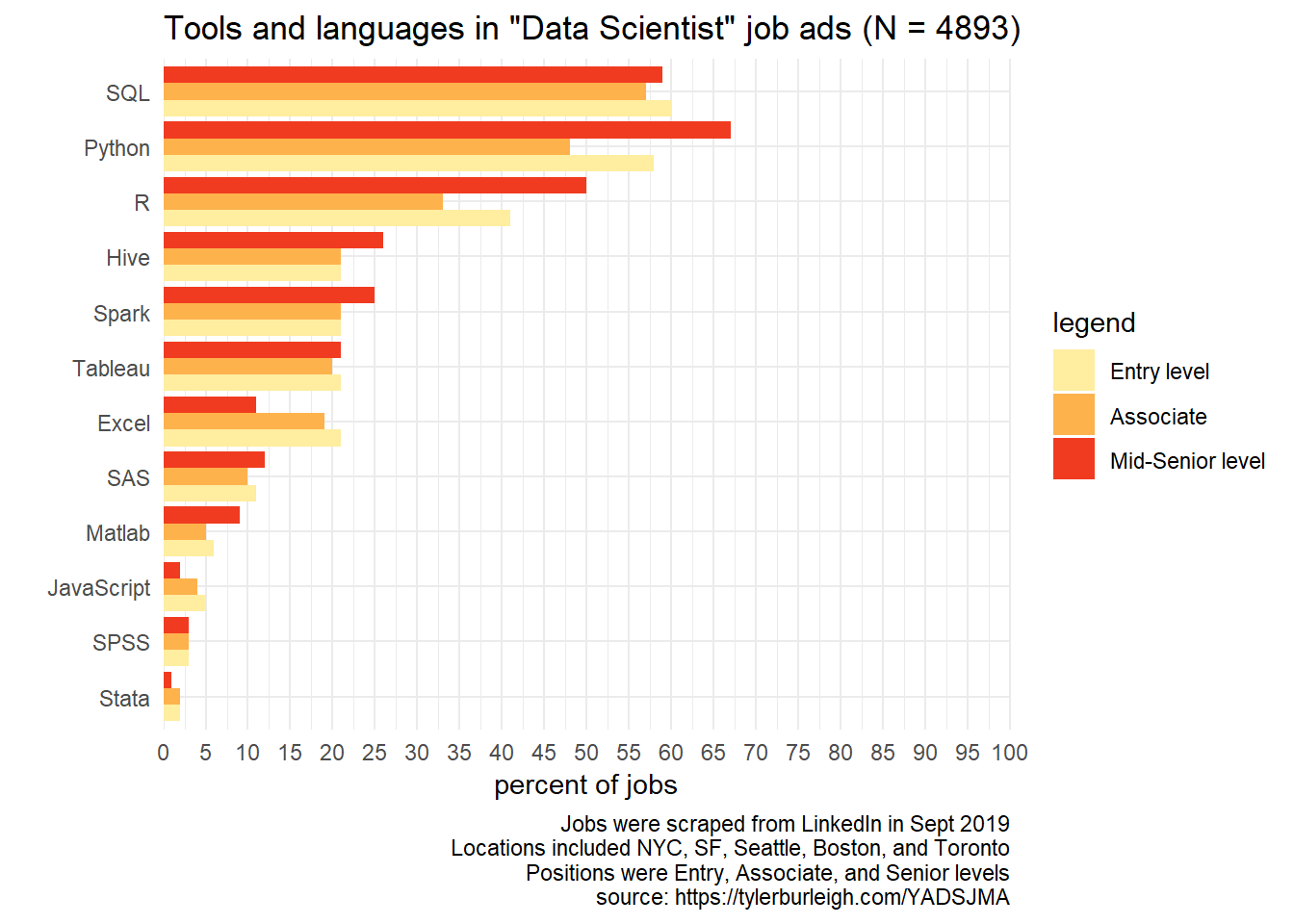Code for the above graph:

``````descriptions %>%
filter(position == ds_filter) %>%
summarize_tools() %>%
generate_barplot(., "Data Scientist", 'Tools and languages', n_ads, 1)``````

#### Other skills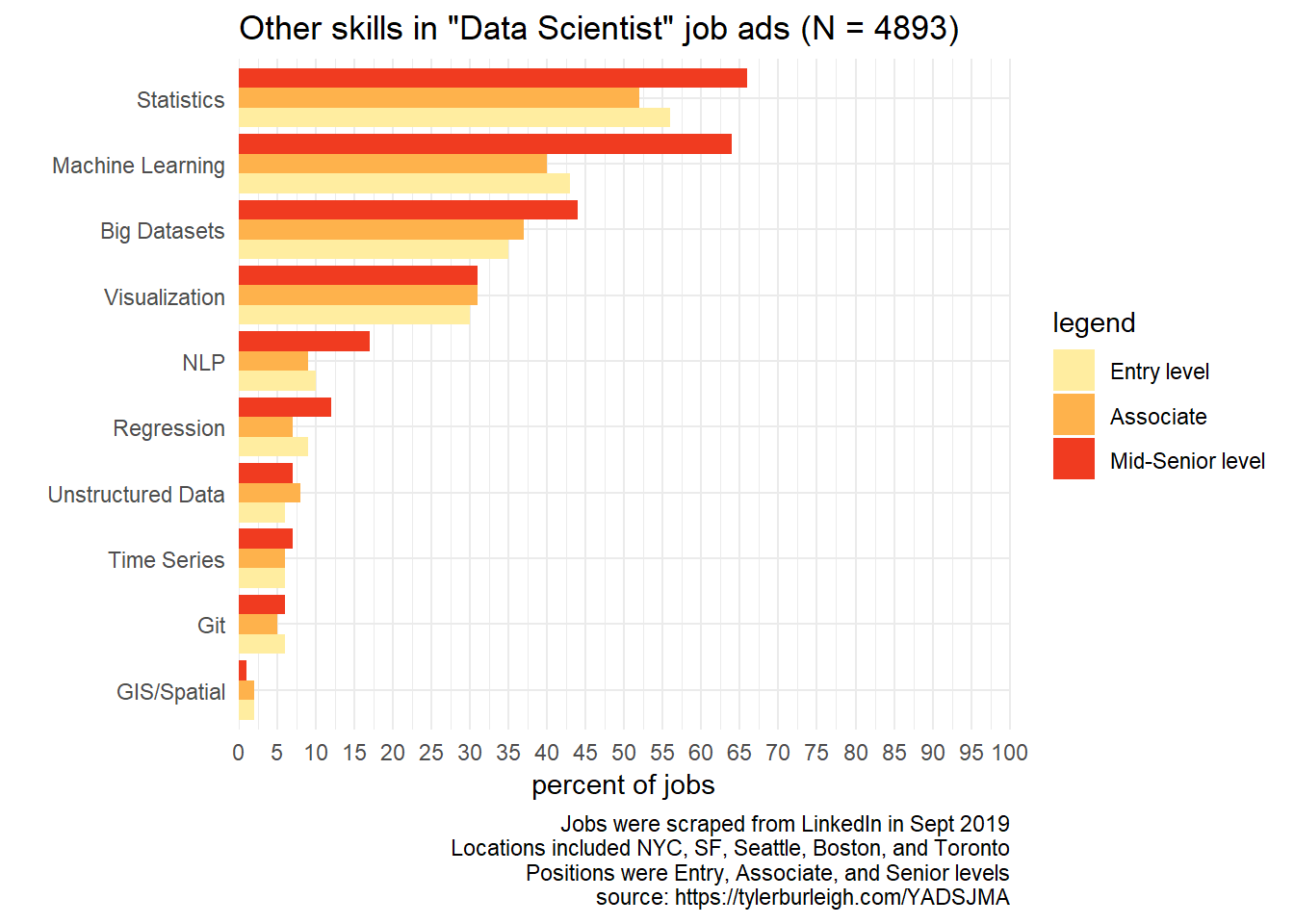Code for the graph above:

``````descriptions %>%
filter(position == ds_filter) %>%
summarize_other_skills() %>%
generate_barplot(., "Data Scientist", 'Other skills', n_ads, 1)``````

#### Degrees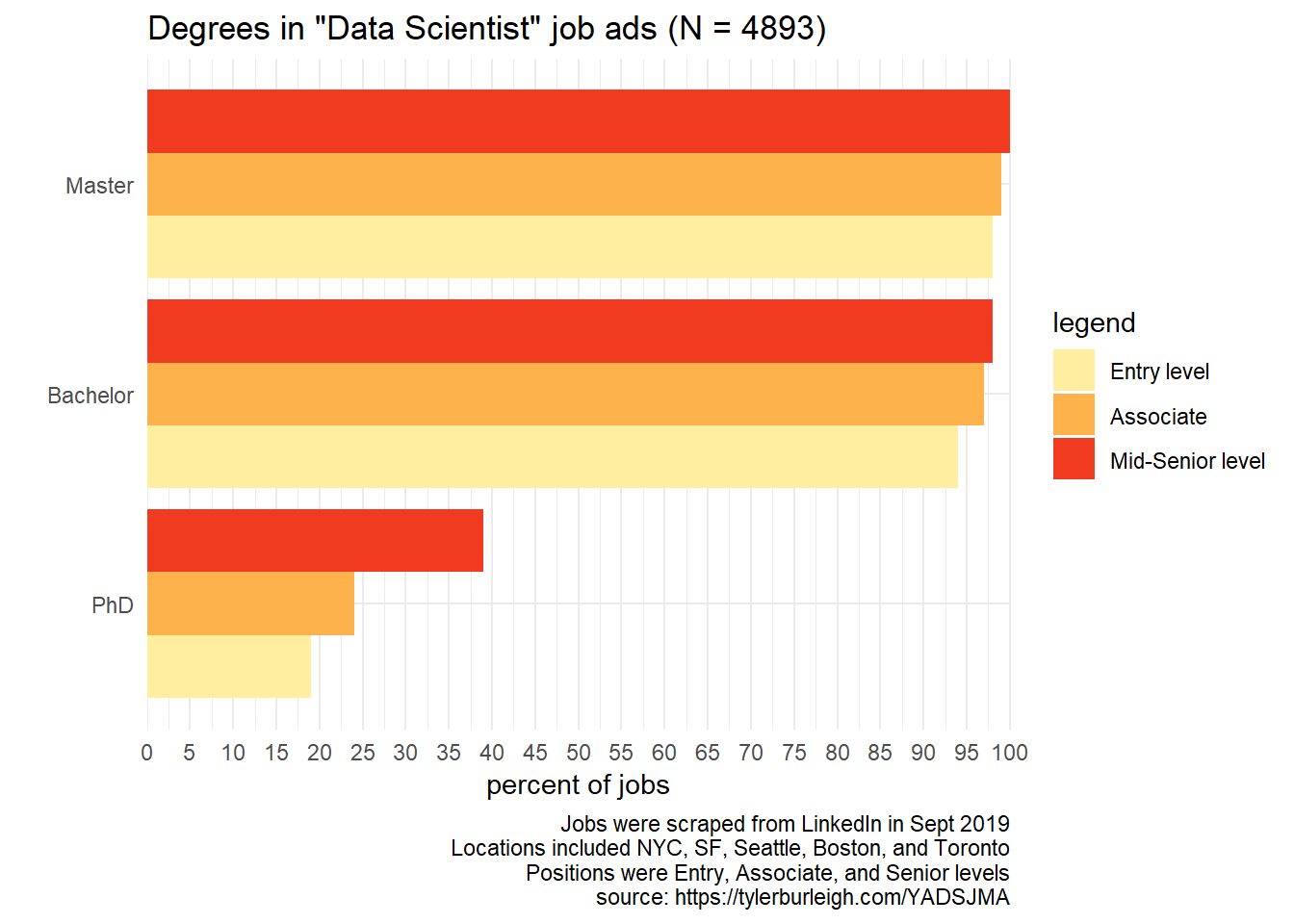Code for the graph above:

``````descriptions %>%
filter(position == ds_filter) %>%
summarize_degrees() %>%
generate_barplot(., "Data Scientist", 'Degrees', n_ads, 1)``````

#### Disciplines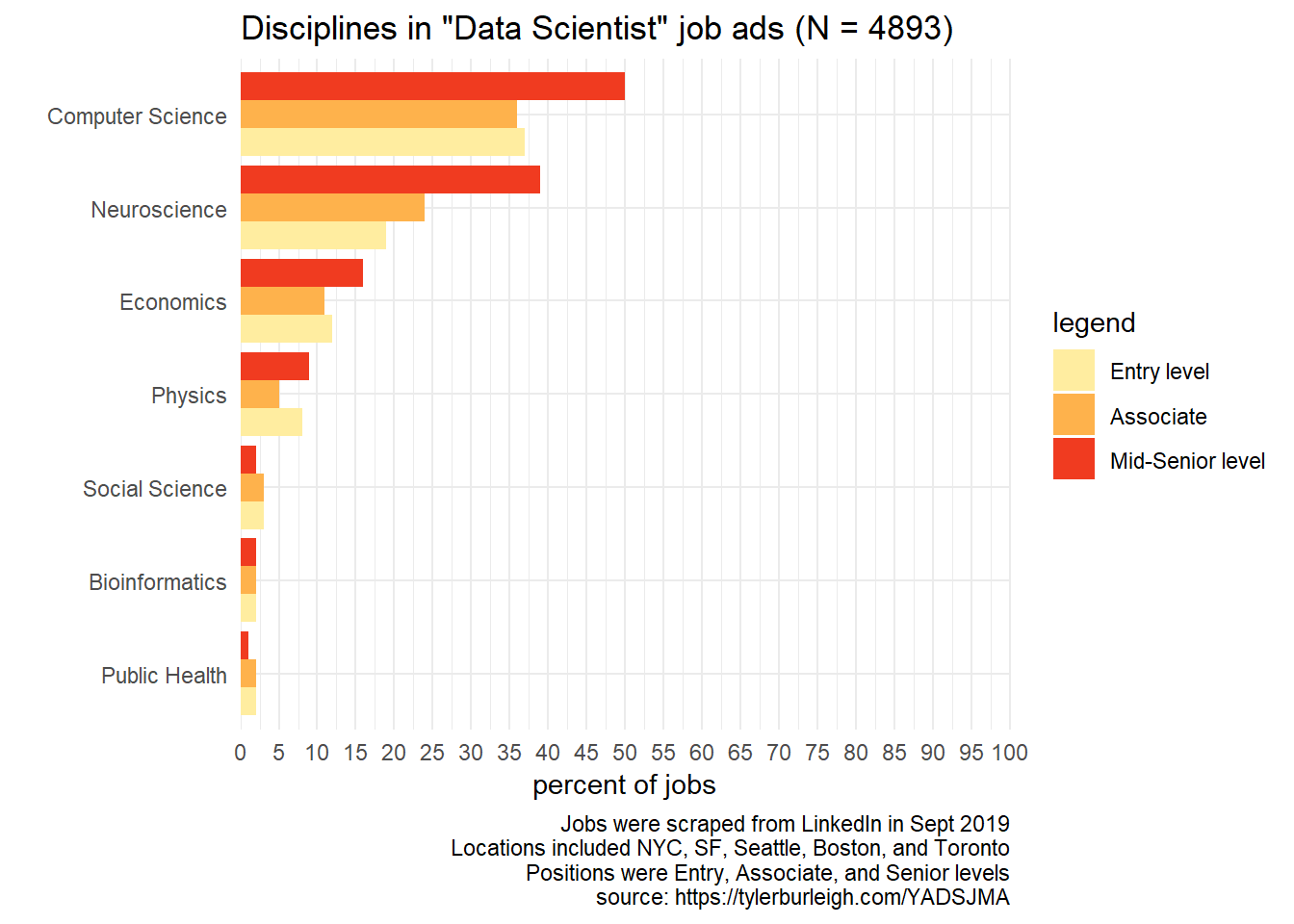Code for the graph above:

``````descriptions %>%
filter(position == ds_filter) %>%
summarize_disciplines() %>%
generate_barplot(., "Data Scientist", 'Disciplines', n_ads, 1)``````

## Toronto

### Toronto

292 data scientist job ads were included in this analysis.

#### Tools / languages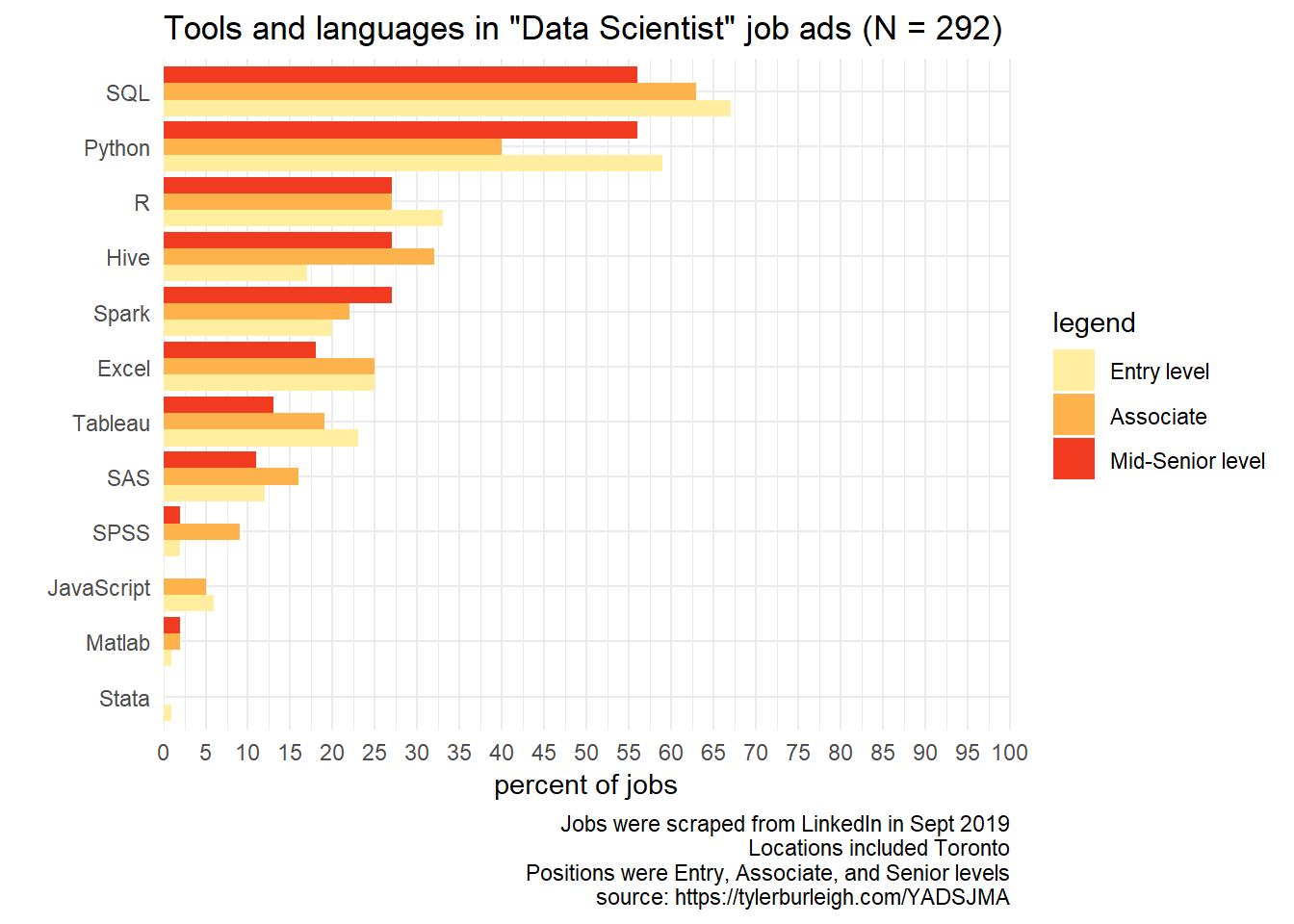Code for the above graph:

``````descriptions %>%
filter(position == ds_filter, location_abbr == "TO") %>%
summarize_tools() %>%
generate_barplot(., "Data Scientist", 'Tools and languages', n_ads, 2)``````

#### Other skills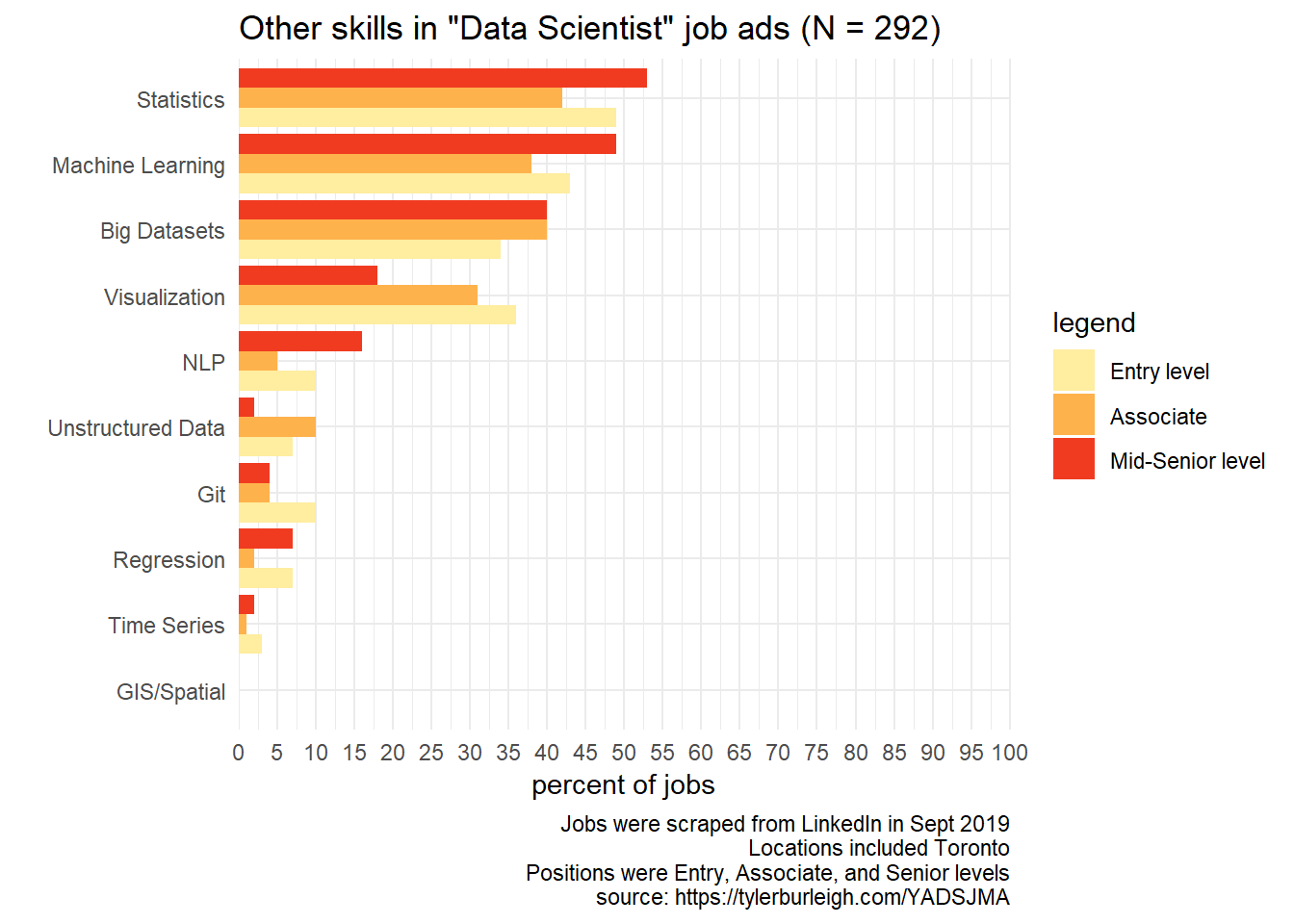Code for the graph above:

``````descriptions %>%
filter(position == ds_filter, location_abbr == "TO") %>%
summarize_other_skills() %>%
generate_barplot(., "Data Scientist", 'Other skills', n_ads, 2)``````

#### Degrees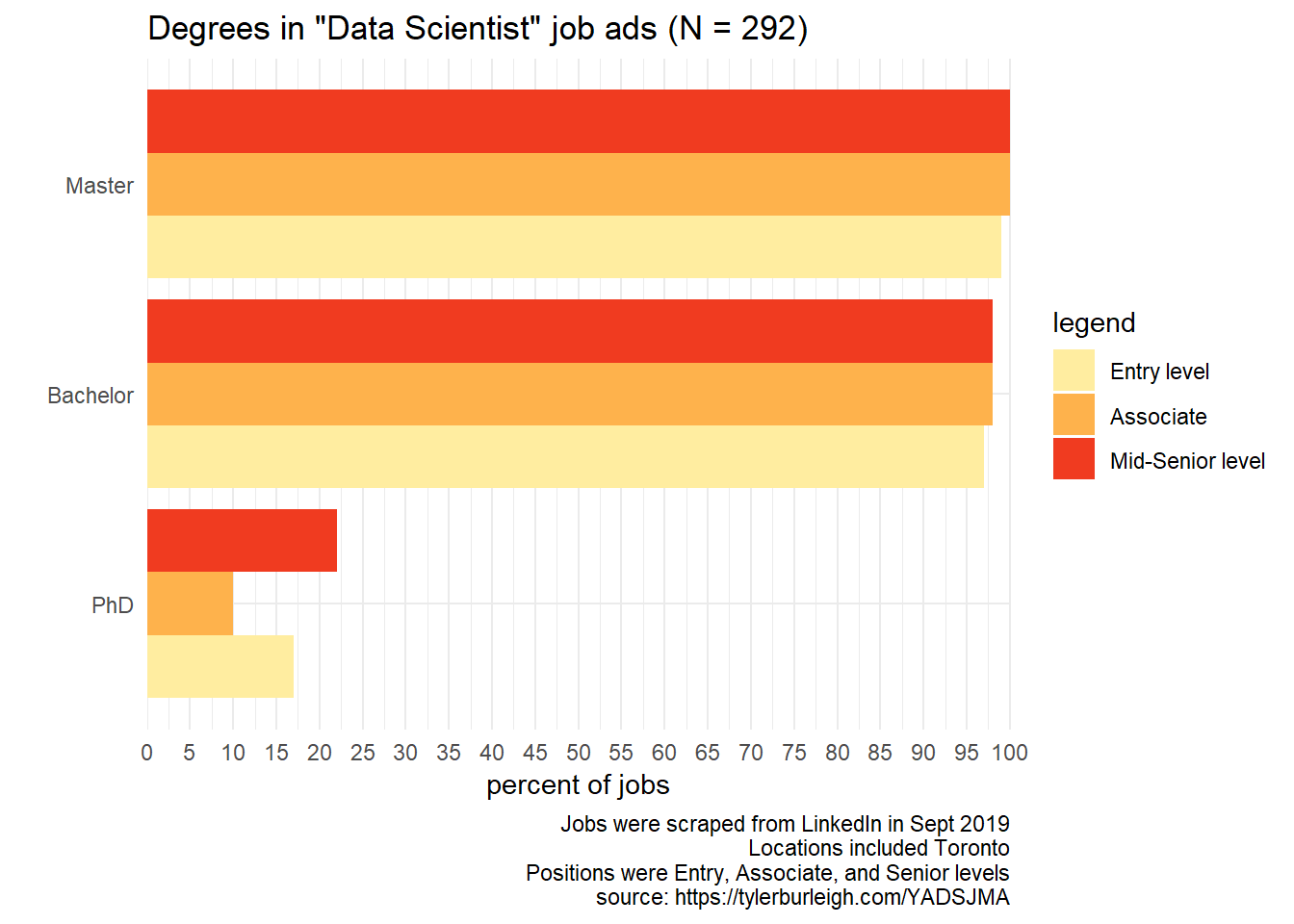Code for the graph above:

``````descriptions %>%
filter(position == ds_filter, location_abbr == "TO") %>%
summarize_degrees() %>%
generate_barplot(., "Data Scientist", 'Degrees', n_ads, 2)``````

#### Disciplines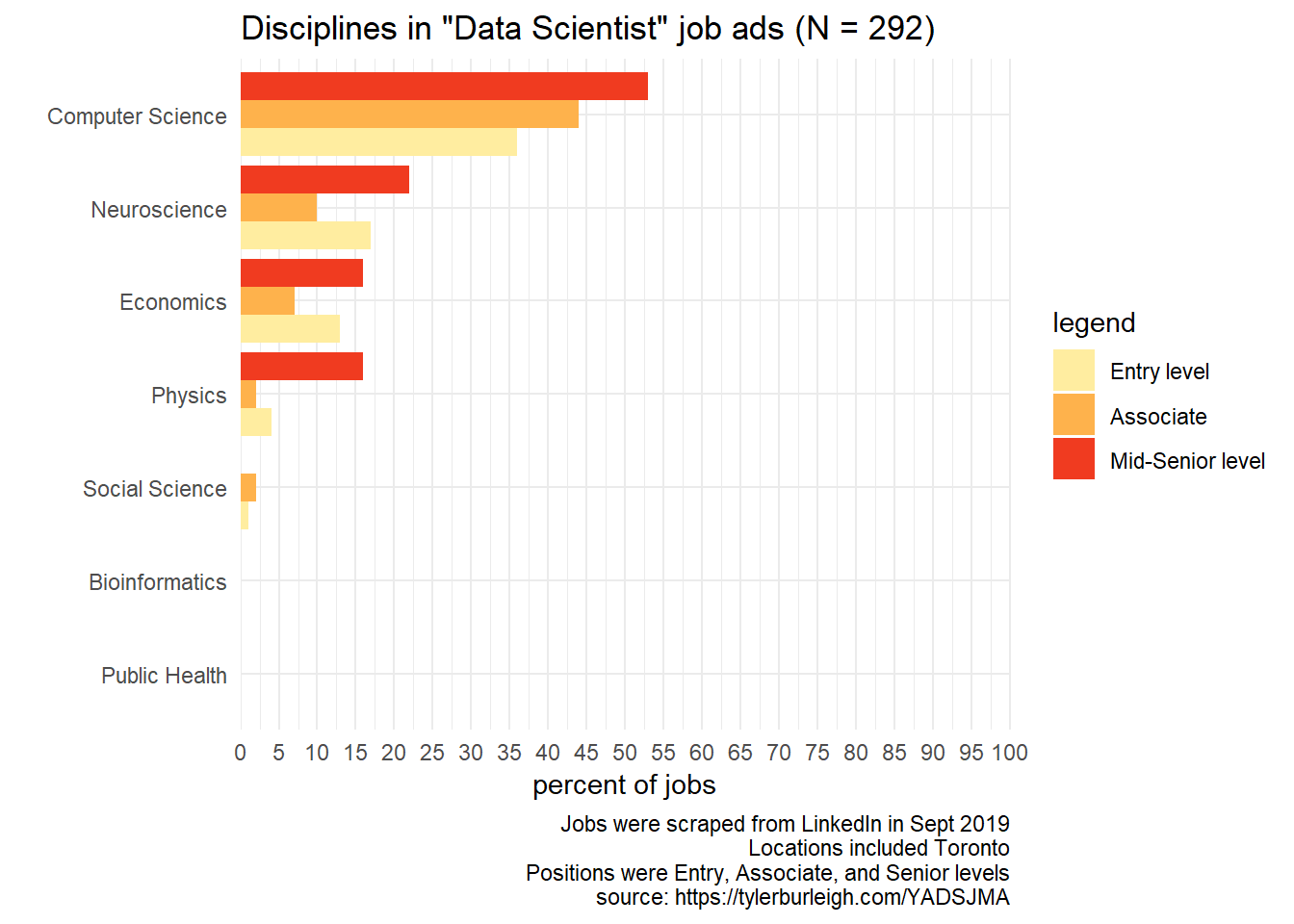Code for the graph above:

``````descriptions %>%
filter(position == ds_filter, location_abbr == "TO") %>%
summarize_disciplines() %>%
generate_barplot(., "Data Scientist", 'Disciplines', n_ads, 2)``````

## Remote option

### Remote option

158 data scientist job ads were included in this analysis.

#### Tools / languages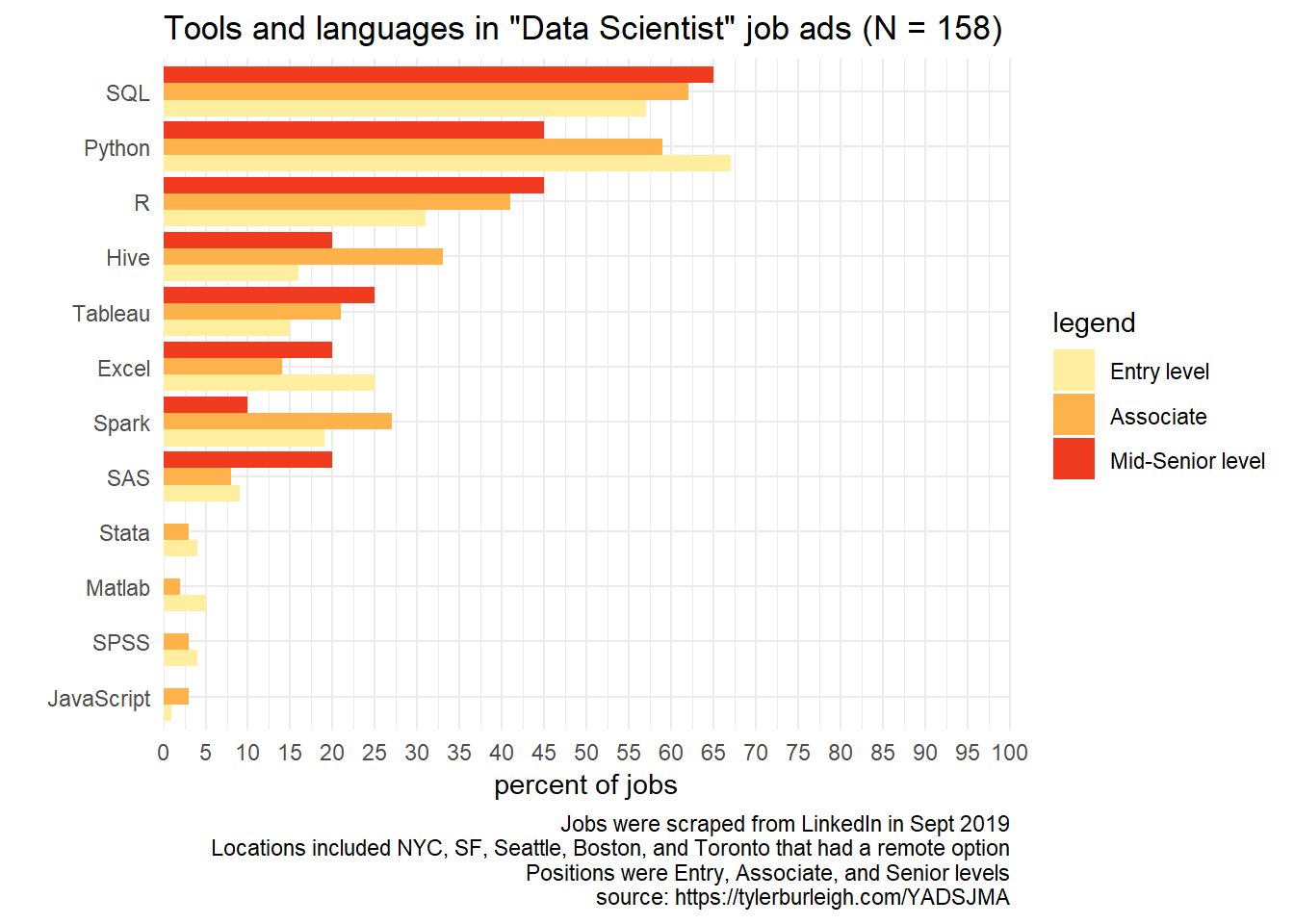Code for the above graph:

``````descriptions %>%
filter(position == ds_filter, remote) %>%
summarize_tools() %>%
generate_barplot(., "Data Scientist", 'Tools and languages', n_ads, 3)``````

#### Other skills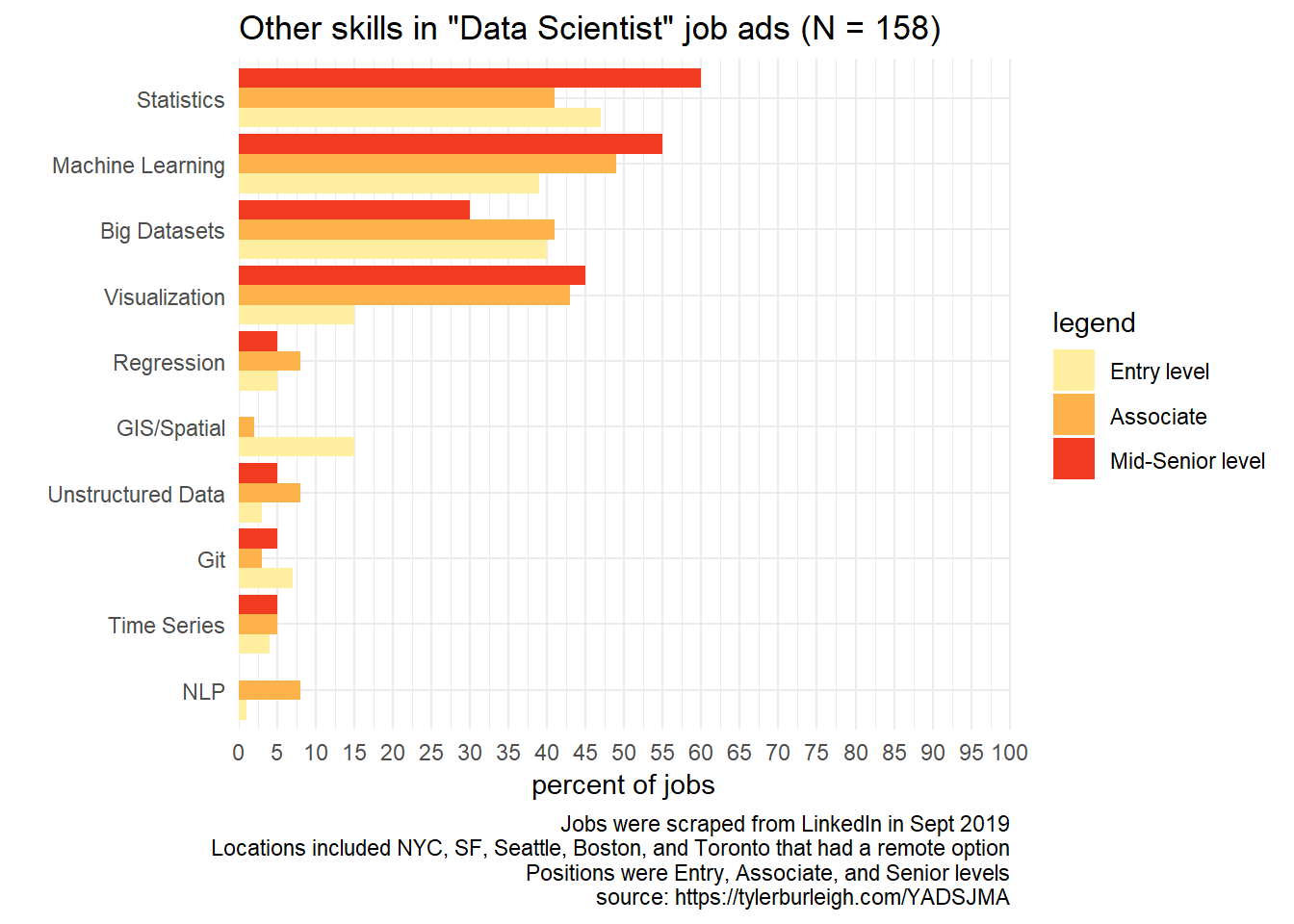Code for the graph above:

``````descriptions %>%
filter(position == ds_filter, remote) %>%
summarize_other_skills() %>%
generate_barplot(., "Data Scientist", 'Other skills', n_ads, 3)``````

#### Degrees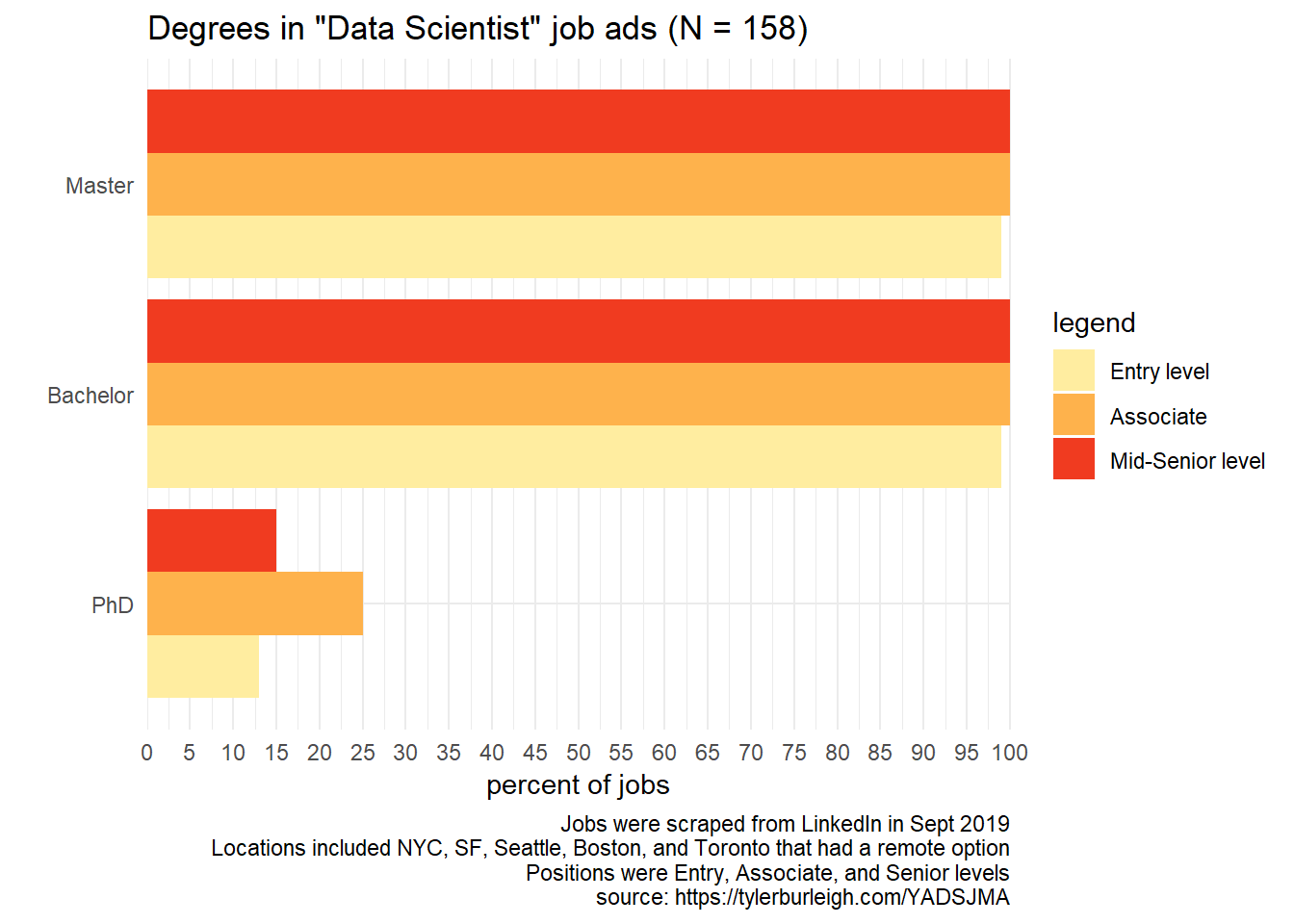Code for the graph above:

``````descriptions %>%
filter(position == ds_filter, remote) %>%
summarize_degrees() %>%
generate_barplot(., "Data Scientist", 'Degrees', n_ads, 3)``````

#### Disciplines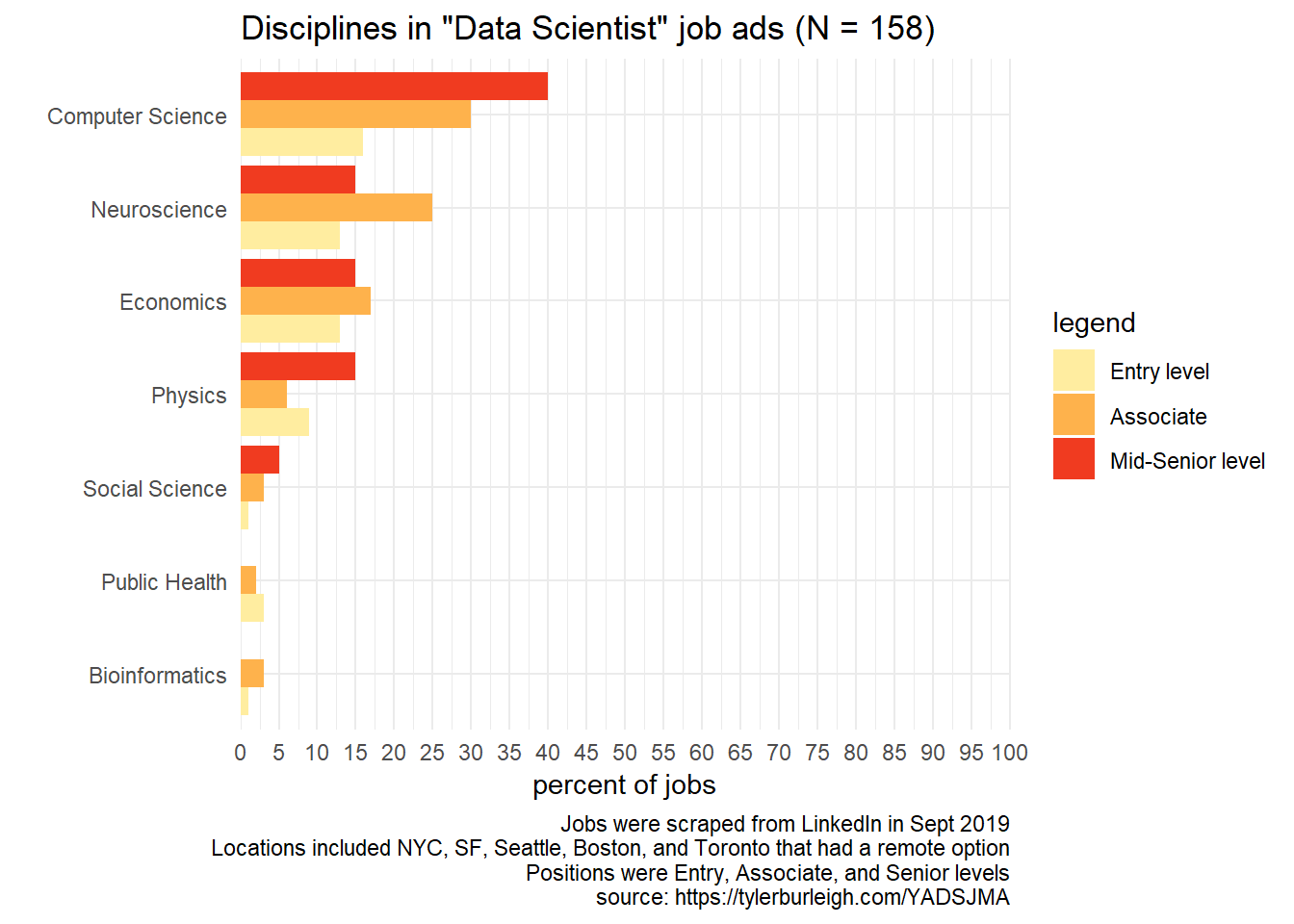Code for the graph above:

``````descriptions %>%
filter(position == ds_filter, remote) %>%
summarize_disciplines() %>%
generate_barplot(., "Data Scientist", 'Disciplines', n_ads, 3)``````

# Data Analyst jobs

## All locations

### All locations

3340 data analyst job ads were included in this analysis.